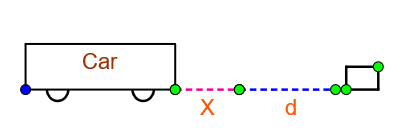QuestionAnswers

# A driver takes $0.20s$ to apply the brakes after he sees a need for it. This is called the reaction time of the driver. If he is driving a car at a speed of 54 km/h and the brakes cause a deceleration of $6.0m/{{s}^{2}}$, find the distance travelled by the car after he sees the need to put the brakes on.

Hint: Here we are given reaction time, initial velocity of the car and its deceleration time. We have to find the stopping distance after the application of break by the driver. To find this we use the equations of motion. By using the first kinematic equation we get the time taken for the car to stop after applying break, and thereby we get the total time taken by the car to stop. Applying all the known variables in the third kinematic equation we get the distance.

Formula used:
\begin{align} & v=u+at \\ & s=ut+\dfrac{1}{2}a{{t}^{2}} \\ \end{align}

In the question we have a driver driving a car.Consider the car and obstacle at a distance x + d from the car.
Let the distance travelled by the car before the driver applies break be ‘x’
Let the distance travelled by the car after applying break be ‘d’.
The reaction time taken by the driver after seeing an obstacle be ‘${{t}_{1}}$’.
${{t}_{1}}=0.20s$
Deceleration caused by the break is =$6.0m/{{s}^{2}}$, since it is deceleration it can written as,
$a=-6.0m/{{s}^{2}}$
Speed at which the driver was driving the car is the initial velocity of the car, which is given as
\begin{align} & u=54km/hr \\ & u=54\times \dfrac{5}{18}m/s \\ & u=15m/s \\ \end{align}
The distance the travelled by car during 0.20s, i.e. distance ‘x’ will be
$x=ut$, Since the car was moving with constant speed.
Substituting the values of u and t, we get x as
\begin{align} & x=15\times 0.2 \\ & x=3m \\ \end{align}
I.e. The car travels a distance of 3m before applying break.
We need to find the distance the car travels after the application of the brake.
We know that, when the driver applies the brake and comes to a stop, its final velocity will become zero.
$v=0m/s$
We have initial velocity,
$u=15m/s$
Deceleration,
$a=-6.0m/{{s}^{2}}$
Now let us apply the first kinematic equation
$v=u+at$, Where $'v'$ is final velocity, $'u'$ is initial velocity, $'a'$ is deceleration and $'t'$ is the time.
By solving this we will get the time taken for the car to stop after applying brake i.e. the deceleration time.
Let this time be ${{t}_{2}}$
Then,
\begin{align} & {{t}_{2}}=\dfrac{v-u}{a} \\ & {{t}_{2}}=\dfrac{0-15}{-6} \\ & {{t}_{2}}=2.5s \\ \end{align}
Therefore the total time = reaction time + deceleration time
Total time,
\begin{align} & t={{t}_{1}}+{{t}_{2}} \\ & t=0.2+2.5 \\ & t=2.7s \\ \end{align}
Now to find the stopping distance, let us apply the third equation of motion,
\begin{align} & s=ut+\dfrac{1}{2}a{{t}^{2}} \\ & s=\left( 15\times 2.7 \right)+\dfrac{1}{2}\left( -6 \right)\left( {{2.7}^{2}} \right) \\ & s=40.5-21.87 \\ & s=18.63m \\ \end{align}
Therefore distance travelled by car after the driver sees the need to apply break = 18.63m.

So, the correct answer is “Option A”.

Note:
Equations of motion are used to derive components like displacement, velocity, acceleration and time. There are three equations of motion:
\begin{align} & 1.v=u+at \\ & 2.{{v}^{2}}={{u}^{2}}+2as \\ & 3.s=ut+\dfrac{1}{2}a{{t}^{2}} \\ \end{align}
Reaction time is the minimum duration a person takes to respond to stimuli.
Deceleration is negative acceleration. When a body is slowing down, reducing its speed then the rate at which the speed is reduced is called deceleration.
To convert the time in $km/hr$ to$m/s$ , we multiply the value in $km/hr$ with$\dfrac{5}{18}$
$1m/s=\dfrac{5}{18}km/hr$.